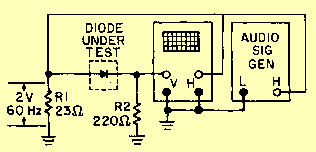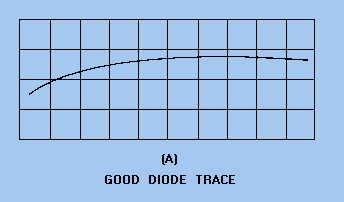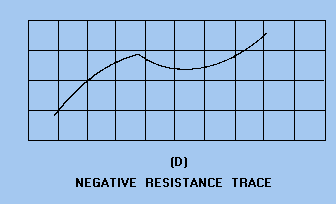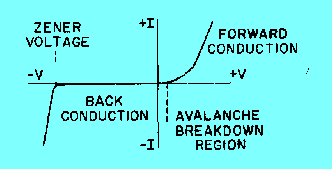Diode testersCustom SearchDiodes, such as general-purpose germanium and silicon diodes, power silicon diodes, and microwave silicon diodes, are most effectively tested under actual operating conditions. However, rectifier testers are available for you to determine direct-current characteristics that provide an indication of diode quality. Rf Diode Test A common type of diode test set is a combination ohmmeter-ammeter. You can make measurements of forward resistance, back resistance, and reverse current with this equipment. You can determine the condition of the rectifier under test by comparing its actual values with typical values obtained from test information furnished with the test set or from the manufacturer's data sheets. Comparing the diode's back and forward resistance at a specified voltage provides you with a rough indication of the rectifying property of a diode. A typical back-to-forward resistance ratio is on the order of 10 to 1, and a forward-resistance value of 50 to 80 ohms is common. Switching Diode Test To effectively test diodes used for computer applications, you must obtain back-resistance measurements at a large number of different voltage levels. This can be done efficiently by using a dynamic diode tester in conjunction with an oscilloscope, which is used to display the diode's back-current-versus-voltage curve. You can easily interpret diode characteristics, such as flutter, hysteresis, and negative resistance, through use of the dynamic current and voltage display. You can use an oscilloscope to graphically display the forward- and back-resistance characteristics of a diode. A test circuit used in conjunction with an oscilloscope is shown in figure 2-7. This circuit uses an audio-signal generator as the test signal. It should be adjusted for an approximate 2-volt, 60-hertz signal, as measured across R1. Figure 2-7. - Display circuit used with an oscilloscope.The test signal you apply to the diode is also connected to the horizontal input of the oscilloscope. The horizontal sweep will then display the voltage applied to the diode under test. The voltage developed across current-measuring resistor R2 is applied to the vertical input of the oscilloscope. Since this voltage is proportional to the current through the diode under test, the vertical deflection will indicate diode current. The resulting oscilloscope trace will be similar to the curve shown in figure 2-8. Figure 2-8. - Typical characteristic curve of a silicone diode.Reverse Voltage-Current Analysis You can make an analysis of the reverse voltage-current portion of the characteristic curve for a diode with the method described above or with a diode test set. This test is very important for diodes used in computer applications, where stability of operation is essential. Various diode conditions that may be detected by this test are shown in figure 2-9, view A, view B, view C, and view D. Figure 2-9A. - Diode reverse current-voltage characteristics. GOOD DIODE TRACEFigure 2-9B. - Diode reverse current-voltage characteristics. HYSTERESIS CHARACTERISTICFigure 2-9C. - Diode reverse current-voltage characteristics. FLUTTER (OR DRIFT) CHARACTERISTICFigure 2-9D. - Diode reverse current-voltage characteristics. NEGATIVE RESISTANCE TRACEZener Diode Test An audio signal generator may not be able to produce a high enough voltage for you to test Zener diodes. You can, however, make this test with a diode test set or with the circuit shown in figure 2-10. In this circuit, R1 is used to adjust the input voltage to a suitable value for the Zener diode being tested. Resistor R2 limits the current through the diode. The signal voltage applied to the diode is also connected to the horizontal input of the oscilloscope. The voltage developed across current-measuring resistor R3 is applied to the vertical input of the oscilloscope. The horizontal sweep will therefore represent the applied voltage, and the vertical deflection will indicate the current through the diode under test. Figure 2-11 shows the characteristic pattern of a Zener diode (note the sharp increase in current at the avalanche breakdown point). For the Zener diode to be acceptable, this voltage must be within the limits specified by the manufacturer. Figure 2-10. - Zener diode test circuit.Figure 2-11. - Zener diode characteristic pattern.STATIC RESISTANCE MEASUREMENTS One convenient method of testing a diode requires only your ohmmeter. The forward and back resistances can be measured at a voltage determined by the battery potential of the ohmmeter and the resistance range at which the meter is set. When the test leads of the ohmmeter are connected to the diode, a resistance will be measured that is different from the resistance indicated if the leads are reversed. The smaller value is called the forward resistance, and the larger value is called the back resistance. If the ratio of back-to-forward resistance is greater than 10 to 1, the diode should be capable of functioning as a rectifier. This is a very limited test, which does not take into account the action of the diode at voltages of different magnitudes and frequencies. Some diodes may be damaged by the excessive current produced by some range settings of a standard multimeter. Therefore, you should use a digital multimeter when performing this measurement. Q.14 As a rule of thumb, what is an acceptable ratio of back-to-forward resistance for a diode?Integrated Publishing, Inc. - A (SDVOSB) Service Disabled Veteran Owned Small Business